# 1 Background

Over the last decade, we have seen exponential growth of the scale of scRNA-seq datasets to millions of cells sequenced in a single study. This has enabled researchers to characterize the gene expression profiles of various cell types across tissues. The rapid growth of scRNA-seq data has also created an unique set of challenges, for instance, there is a pressing need for scalable approaches for scRNA-seq data visualization.

This vignette introduces scBubbletree, a transparent workflow for quantitative exploration of single cell RNA-seq data.

In short, the algorithm of scBubbletree performs clustering to identify clusters (bubbles) of transcriptionally similar cells, and then visualizes these clusters as leafs in a hierarchical dendrogram (bubbletree) which describes their natural relationships. The workflow comprises four steps: 1. determining the clustering resolution, 2. clustering, 3. hierarchical cluster grouping and 4. visualization. We explain each step in the following using real scRNA-seq dataset of five cancer cell lines.

To run this vignette we need to load a few R-packages:

library(scBubbletree)
library(ggplot2)
library(ggtree)
library(patchwork)

# 2 Data111 Tian, Luyi, et al. “Benchmarking single cell RNA-sequencing analysis pipelines using mixture control experiments.” Nature methods 16.6 (2019): 479-487.

Here we will analyze a scRNA-seq dataset containing a mixture of 3,918 cells from five human lung adenocarcinoma cell lines (HCC827, H1975, A549, H838 and H2228). The dataset is available here2 https://github.com/LuyiTian/sc_mixology/blob/master/data/ sincell_with_class_5cl.RData.

The library has been prepared with 10x Chromium platform and sequenced with Illumina NextSeq 500 platform. Raw data has been processed with Cellranger. The tool demuxlet has been used to predict the identity of each cell based on known genetic differences between the different cell lines.

# 3 Data processing

Data processing was performed with R-package Seurat. Gene expressions were normalized with the function SCTransform using default parameters, and principal component analysis (PCA) was performed with function RunPCA based on the 5,000 most variable genes in the dataset identified with the function FindVariableFeatures.

In both datasets we saw that the first 15 principal components capture most of the variance in the data, and the proportion of variance explained by each subsequent principal component was negligible. Thus, we used the single cell projections (embeddings) in 15-dimensional feature space, $$A^{3,918\times 15}$$.

# # This script can be used to generate data("d_ccl", package = "scBubbletree")
#
# # create directory
# dir.create(path = "case_study/")
#
# https://github.com/LuyiTian/sc_mixology/raw/master/data/
#   sincell_with_class_5cl.RData
#
#
# # we are only interested in the 10x data object 'sce_sc_10x_5cl_qc'
# d <- sce_sc_10x_5cl_qc
#
# # remove the remaining objects (cleanup)
# rm(sc_Celseq2_5cl_p1, sc_Celseq2_5cl_p2, sc_Celseq2_5cl_p3, sce_sc_10x_5cl_qc)
#
# # get the meta data for each cell
# meta <- colData(d)[,c("cell_line_demuxlet","non_mt_percent","total_features")]
#
# # create Seurat object from the raw counts and append the meta data to it
# d <- Seurat::CreateSeuratObject(counts = d@assays$data$counts,
#                                 project = '')
#
# # check if all cells are matched between d and meta
# # table(rownames(d@meta.data) == meta@rownames)
# d@meta.data <- cbind(d@meta.data, meta@listData)
#
# # cell type predictions are provided as part of the meta data
# table(d@meta.data$cell_line) # # # select 5,000 most variable genes # d <- Seurat::FindVariableFeatures(object = d, # selection.method = "vst", # nfeatures = 5000) # # # Preprocessing with Seurat: SCT transformation + PCA # d <- SCTransform(object = d, # variable.features.n = 5000) # d <- RunPCA(object = d, # npcs = 50, # features = VariableFeatures(object = d)) # # # perform UMAP + t-SNE # d <- RunUMAP(d, dims = 1:15) # d <- RunTSNE(d, dims = 1:15) # # # save the preprocessed data # save(d, file = "case_study/d.RData") # # # save the PCA matrix 'A', meta data 'm' and # # marker genes matrix 'e' # d <- get(load(file ="case_study/d.RData")) # A <- d@reductions$pca@cell.embeddings[, 1:15]
# m <- d@meta.data
# e <- t(as.matrix(d@assays$SCT@data[ # rownames(d@assays$SCT@data) %in%
#     c("ALDH1A1",
#       "PIP4K2C",
#       "SLPI",
#       "CT45A2",
#       "CD74"), ]))
#
# d_ccl <- list(A = A, m = m, e = e)
# save(d_ccl, file = "data/d_ccl.RData")

Load the processed PCA matrix and the meta data

data("d_ccl", package = "scBubbletree")
A <- d_ccl$A m <- d_ccl$m
e <- d_ccl$e # 4 scBubbletree workflow We will analyze this data with scBubbletree. As first input scBubbletree uses matrix $$A^{n\times f}$$ which represents a low-dimensional projection of the original scRNA-seq data, with $$n$$ rows as cells and $$f$$ columns as low-dimension features. We will use the PCA data generated by Seurat as $$A$$. In particular, we will use the first 15 principal components (PCs) as every additional PC explains negligible amount of variance in the data. Important remark about $$A$$: the scBubbletree workflow works directly with the numeric matrix $$A^{n\times f}$$ and is agnostic to the initial data processing protocol. This enables seamless integration of scBubbletree with computational pipelines using objects generated by the R-packages Seurat and SingleCellExperiment. The users simply have to extract $$A$$ from the corresponding Seurat or SingleCellExperiment objects. # A has n=cells as rows, f=features as columns (e.g. from PCA) dim(A) FALSE  3918 15 The scBubbletree workflow performs the following steps: 1. determine the clustering resolution (clusters $$k$$ or resolution $$r$$) 2. graph-based community detection (e.g. with Louvain) or k-means clustering 3. hierarchical organization of clusters (bubbles) 4. visualization ## 4.1 1. determine the clustering resolution If we use graph-based community detection (recommended for scRNA-seq) with e.g. the Louvain or Leiden method, then we need to find appropriate value for the resolution parameter $$r$$. Otherwise, we can use the simpler k-means clustering algorithm in which case we need to find an appropriate value of the number of clusters $$k$$. ### 4.1.1 1.1 determining the number of clusters $$k$$ How many clusters (cell types) are there are in the data? Can we guess a reasonable value of $$k$$? To find a reasonable value of $$k$$ we can study the literature or databases such as the human protein atlas database (HPA). We can also use the function get_k for data-driven inference of $$k$$ based on the Gap statistic and the within- cluster sum of squares (WCSS). As this is a toy dataset, we will skip the first approach and perform a data-driven search for $$k$$ using get_k. As input we need to provide the matrix $$A$$ as input and a vector of $$k$$s. The output will be the Gap statistic and WCSS estimates for each $$k$$. Lets run get_k now: b_k <- get_k(B_gap = 5, ks = 1:10, x = A, n_start = 50, iter_max = 200, kmeans_algorithm = "MacQueen", cores = 1) The Gap statistic and WCSS curves have a noticeable knee (elbow) at $$k=5$$. Hence, $$k$$=5 appears to be reasonable first choice of $$k$$. Means (points) and 95% confidence intervals are shown for the Gap statistic at each $$k$$ computed using B_gap=5 MCMC simulations. g0 <- ggplot(data = b_k$gap_stats_summary)+
geom_line(aes(x = k, y = gap_mean))+
geom_point(aes(x = k, y = gap_mean), size = 1)+
geom_errorbar(aes(x = k, y = gap_mean, ymin = L95, ymax = H95), width = 0.1)+
ylab(label = "Gap")|
ggplot(data = b_k$wcss_stats_summary)+ geom_line(aes(x = k, y = wcss_mean))+ geom_point(aes(x = k, y = wcss_mean), size = 1)+ ylab(label = "WCSS")+ scale_y_log10()+ annotation_logticks(base = 10, sides = "l") g0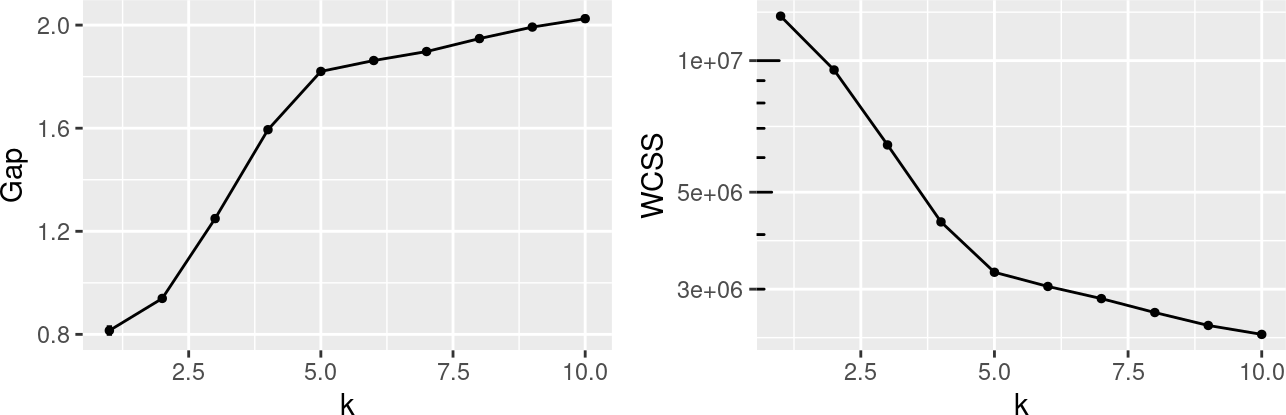### 4.1.2 1.2 determining the resolution parameter $$r$$ For Louvain clustering we need to select a clustering resolution $$r$$. Higher resolutions lead to more communities and lower resolutions lead to fewer communities. We can use the same strategy as before to find a reasonable reasonable value of $$r$$. Lets use the function get_r for data-driven estimation of $$r$$ based on the Gap statistic and WCSS. As input we need to provide the matrix $$A$$ and a vector of $$r$$s. The output will be the Gap statistic and WCSS estimate for each $$r$$ (or the number of communities $$k'$$ detected at resolution $$r$$). b_r <- get_r(B_gap = 5, rs = 10^seq(from = -4, to = 0, by = 0.35), x = A, n_start = 10, iter_max = 50, algorithm = "original", knn_k = 50, cores = 1) The Gap statistic and WCSS curves have noticeable knees (elbows) at $$k'=5$$ ($$r=0.0025$$). Means (points) and 95% confidence intervals are shown for the Gap statistic at each $$k$$ computed using B_gap=5 MCMC simulations. g0_r <- (ggplot(data = b_r$gap_stats_summary)+
geom_line(aes(x = k, y = gap_mean))+
geom_point(aes(x = k, y = gap_mean), size = 1)+
geom_errorbar(aes(x = k, y = gap_mean, ymin = L95, ymax = H95), width = 0.1)+
ylab(label = "Gap")+
xlab(label = "k'")|
ggplot(data = b_r$gap_stats_summary)+ geom_line(aes(x = r, y = gap_mean))+ geom_point(aes(x = r, y = gap_mean), size = 1)+ geom_errorbar(aes(x = r, y = gap_mean, ymin = L95, ymax = H95), width = 0.1)+ ylab(label = "Gap")+ xlab(label = "r")+ scale_x_log10()+ annotation_logticks(base = 10, sides = "b"))/ (ggplot(data = b_r$wcss_stats_summary)+
geom_line(aes(x = k, y = wcss_mean))+
geom_point(aes(x = k, y = wcss_mean), size = 1)+
ylab(label = "WCSS")+
xlab(label = "k'")|
ggplot(data = b_r$wcss_stats_summary)+ geom_line(aes(x = r, y = wcss_mean))+ geom_point(aes(x = r, y = wcss_mean), size = 1)+ ylab(label = "WCSS")+ xlab(label = "r")+ scale_x_log10()+ annotation_logticks(base = 10, sides = "b")) g0_r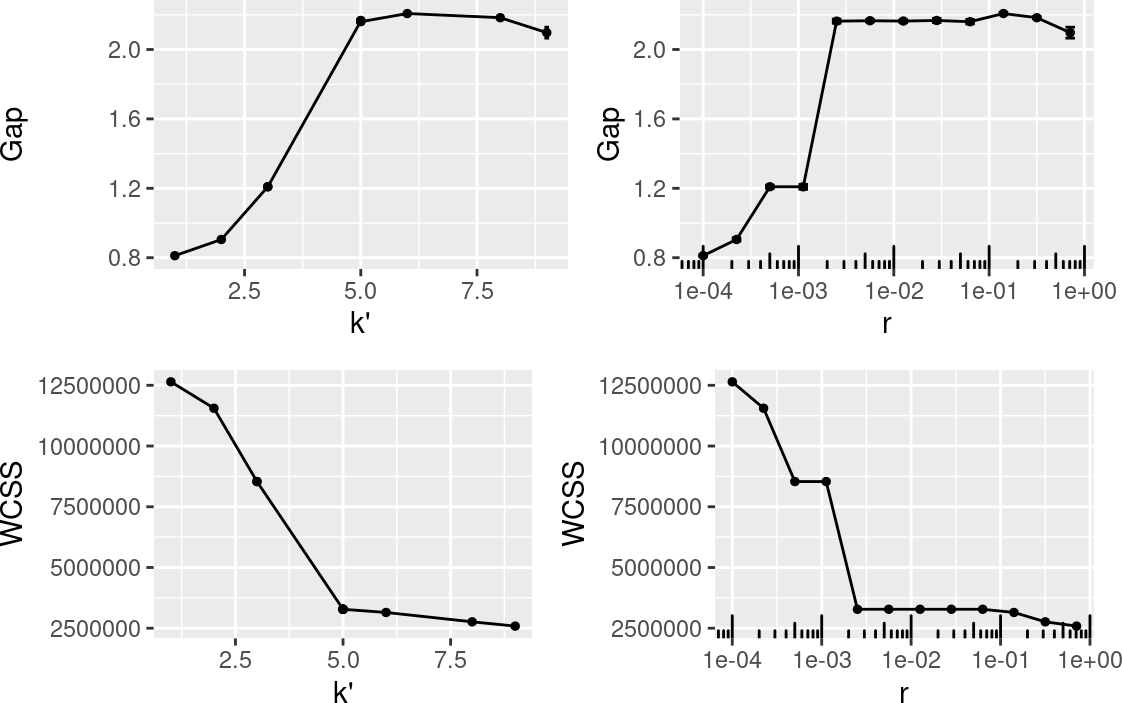A range of resolutions yields $$k=5$$ number of communities, i.e. $$r = 0.0025$$ and $$r = 0.125$$ result in $$k=5$$. Lets use $$r=0.1$$ for clustering ggplot(data = b_r$gap_stats_summary)+
geom_point(aes(x = r, y = k), size = 1)+
xlab(label = "r")+
ylab(label = "k'")+
scale_x_log10()+
annotation_logticks(base = 10, sides = "b")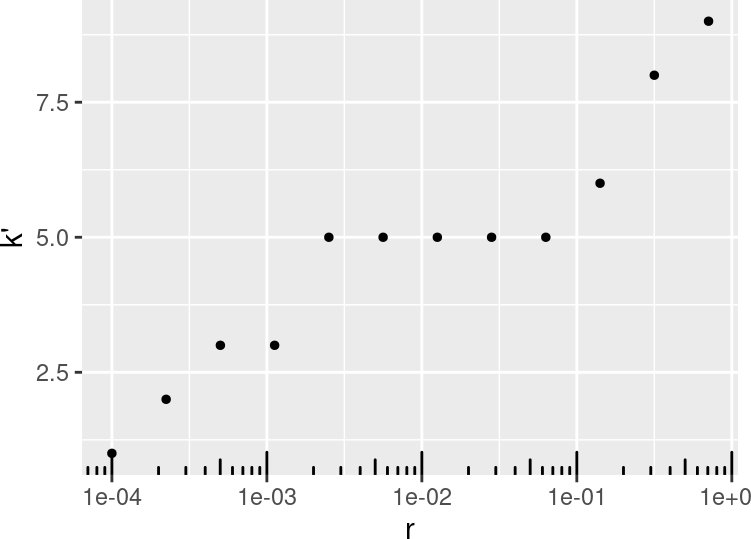knitr::kable(x = b_r$gap_stats_summary[b_r$gap_stats_summary$k == 5, ], digits = 4, row.names = FALSE) gap_mean r k gap_SE L95 H95 2.1634 0.0025 5 0.0060 2.1517 2.1751 2.1660 0.0056 5 0.0036 2.1590 2.1730 2.1640 0.0126 5 0.0041 2.1560 2.1720 2.1674 0.0282 5 0.0059 2.1559 2.1789 2.1603 0.0631 5 0.0063 2.1479 2.1726 ## 4.2 2. clustering ### 4.2.1 2.1 with k-means Now that we found out that $$k=5$$ is a reasonable choice based on the data, we will perform k-means clustering with $$k=5$$ and $$A$$ as inputs. For this we will use the function kmeans (R-package stats) which offers various variants of k-means. Here we will use MacQueen’s k-means variant and perform $$n_\textit{start} = 1000$$ (default in scBubbletree) random starts and a maximum number of iterations $$iter_\textit{max}=300$$. Important remark: for smaller datasets (e.g. $$n<50,000$$) $$n_{start}=1000$$ and $$n_{iter} = 300$$ are unnecessarily high, however for larger datasets this is necessary to make sure that k-means converges. ## 4.3 3. hierarchical grouping After the clustering is complete we will organize the bubbles in a natural hierarchy. For this we perform $$B$$ bootstrap iterations (default $$B=200$$). In iteration $$b$$ the algorithm draws a random subset of $$N_\textit{eff}$$ (default $$N_\textit{eff}=200$$) cells with replacement from each cluster and computes the average inter-cluster Euclidean distances. This data is used to populate the distance matrix ($$D^{k\times k}_b$$), which is provided as input for hierarchical clustering with average linkage to generate a hierarchical clustering dendrogram $$H_b$$. The collection of distance matrices that are computed during $$B$$ iterations are used to compute a consensus (average) distance matrix ($$\hat{D}^{k\times k}$$) and from this a corresponding consensus hierarchical dendrogram (bubbletree; $$\hat{H}$$) is constructed. The collection of dendrograms are used to quantify the robustness of the bubbletree topology, i.e. to count the number of times each branch in the bubbletree is found among the topologies of the bootstrap dendrograms. Branches can have has variable degrees of support ranging between 0 (no support) and $$B$$ (complete support). Distances between bubbles (inter- bubble relationships) are described quantitatively in the bubbletree as sums of branch lengths. Steps 2.1 and 3. are performed next k5_kmeans <- get_bubbletree_kmeans( x = A, k = 5, cores = 1, B = 200, N_eff = 200, round_digits = 1, show_simple_count = FALSE, kmeans_algorithm = "MacQueen") … and plot the bubbletree k5_kmeans$tree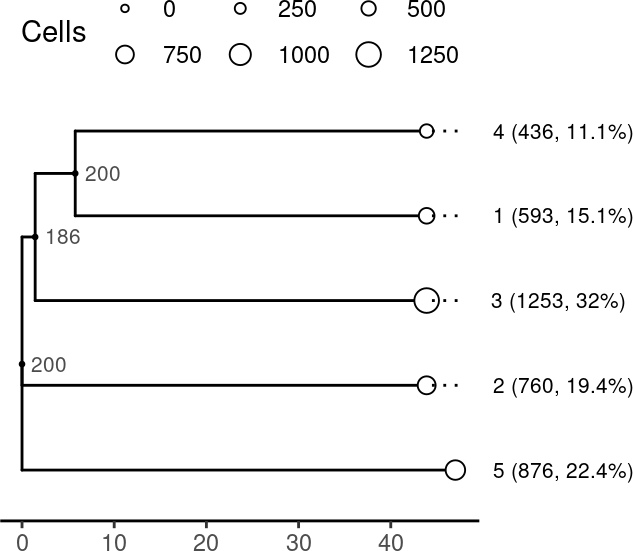Lets describe the bubbletree:

bubbles: The bubbletree has k=5 bubbles (clusters) shown as leaves. The absolute and relative cell frequencies in each bubble and the bubble IDs are shown as labels. Bubble radii scale linearly with absolute cell count in each bubble, i.e. large bubbles have many cells and small bubbles contain few cells.

Bubble 1 is the largest one in the dendrogram and contains 1,253 cells ($$\approx$$ 32% of all cells in the dataset). Bubble 4 is the smallest one and contains only 436 cells ($$\approx$$ 11% of all cells in the dataset).

We can access the bubble data shown in the bubbletree

knitr::kable(k5_kmeans$tree_meta, digits = 2, row.names = FALSE) label Cells n p pct lab_short lab_long tree_order 4 436 3918 0.11 11.1 4 (0.4K, 11.1%) 4 (436, 11.1%) 5 1 593 3918 0.15 15.1 1 (0.6K, 15.1%) 1 (593, 15.1%) 4 3 1253 3918 0.32 32.0 3 (1.3K, 32%) 3 (1253, 32%) 3 2 760 3918 0.19 19.4 2 (0.8K, 19.4%) 2 (760, 19.4%) 2 5 876 3918 0.22 22.4 5 (0.9K, 22.4%) 5 (876, 22.4%) 1 topology: inter-bubble distances are represented by sums of branch lengths in the dendrogram. Branches of the bubbletree are annotated with their bootstrap support values (red branch labels). The branch support value tells us how manytimes a given branch from the bubbletree was found among the $$B$$ bootstrap dendrograms. We ran get_bubbletree_kmeans with $$B=200$$. All but one branch have complete (200 out of 200) support, and one branch has lower support of 179 (85%). This tells us that the branch between bubbles (3, 4) and 1 is not as robust. ### 4.3.1 2.2 with Louvain Lets also perform clustering with the Louvain algorithm (function FindClusters, R-package Seurat) and resolution parameter $$r=0.1$$. There are numerous variants of the Louvain algorithm. Here we will use the original implementation. We will do clustering with $$n_\textit{start} = 20$$ random starts and a maximum number of iterations $$iter_\textit{max} = 100$$. Steps 2.2 and 3. (hierarchical clustering) are performed next k5_louvain <- get_bubbletree_graph(x = A, r = 0.0025, n_start = 20, iter_max = 100, algorithm = "original", knn_k = 50, cores = 1, B = 200, N_eff = 200, round_digits = 1, show_simple_count = FALSE) … and plot the bubbletree. We see nearly identical dendrogram as the one generated by kmeans clustering. The bubble IDs are different but we see similar bubble sizes, topology and branch robustness values. k5_louvain$tree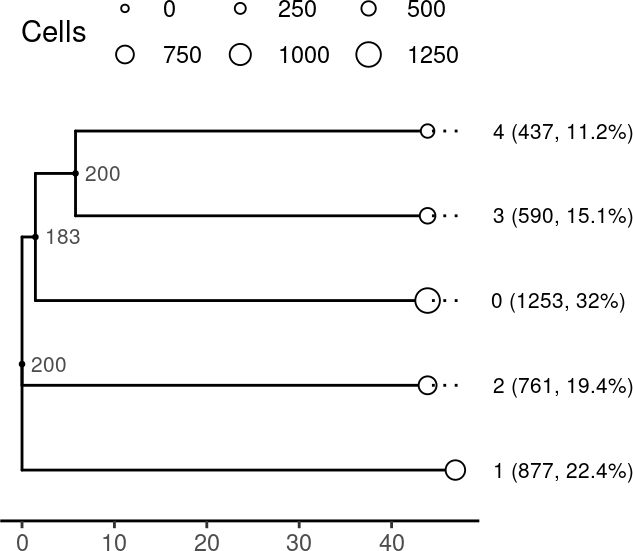The two dendrograms shown side-by-side:

k5_kmeans$tree|k5_louvain$tree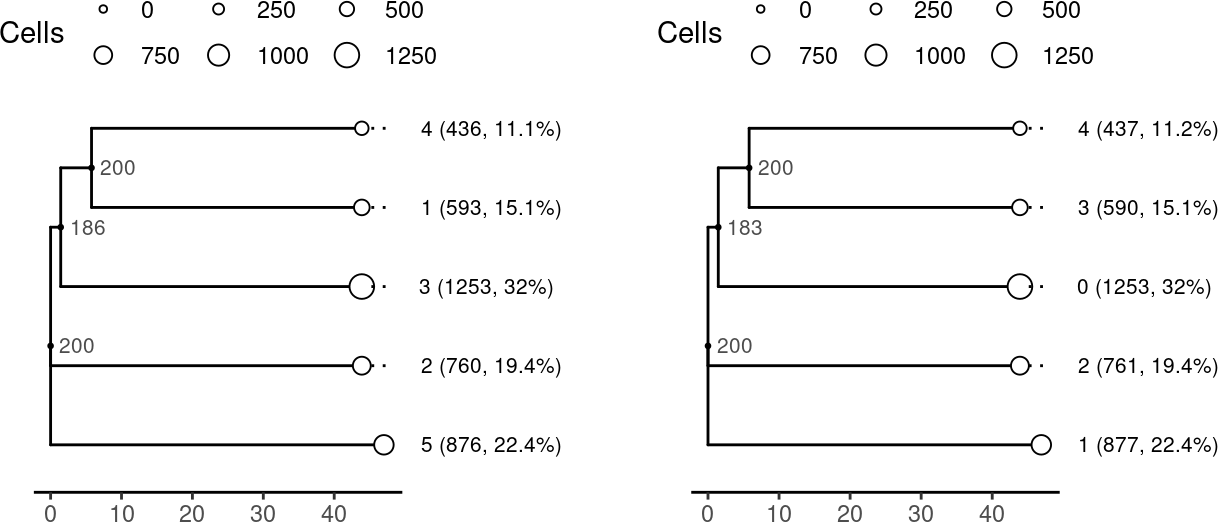Given the high degree of similarity between the two clustering solutions we proceed in the next with the k-means results.

## 4.4 4. visualization

To extract biologically useful information from the bubbletree (and also for 2D UMAP or t-SNE plots) we need to adorn it with biologically relevant cell features. This includes both numeric and categorical cell features.

Numeric cell features:

• gene expression
• % of mitochondrial transcripts
• number of UMIs, genes detected

Categorical cell features:

• cell type label (e.g. B-cells, T-cells, moncytes, …)
• cell cycle phase (e.g. S, M, G1, …)
• sample name (e.g. S1, S2, S3, …)
• treatment group (e.g. cancer vs. control cell)
• multiplet status (e.g. singlet, doublet or multiplet)

In the next two paragraph we will explain how to ‘attach’ numeric and categorical features to the bubbletree using scBubbletree.

## 4.5 Attaching categorical features

Categorical cell features can be ‘attached’ to the bubbletree using the function get_cat_tiles. Here we will show the relative frequency of cell type labels across the bubbles (parameter integrate_vertical=TRUE).

Interpretation of the figure below:

• we see high degree of co-occurrence between cell lines and bubbles, i.e.  each bubble is made up of cells from a distinct cell line
• for instance, 99.8% of cells that have feature HCC827 are found in bubble 2
• columns in the tile plot integrate to 100%
w1 <- get_cat_tiles(btd = k5_kmeans,
f = m$cell_line_demuxlet, integrate_vertical = TRUE, round_digits = 1, x_axis_name = 'Cell line', rotate_x_axis_labels = TRUE, tile_text_size = 2.75) (k5_kmeans$tree|w1$plot)+ patchwork::plot_layout(widths = c(1, 1))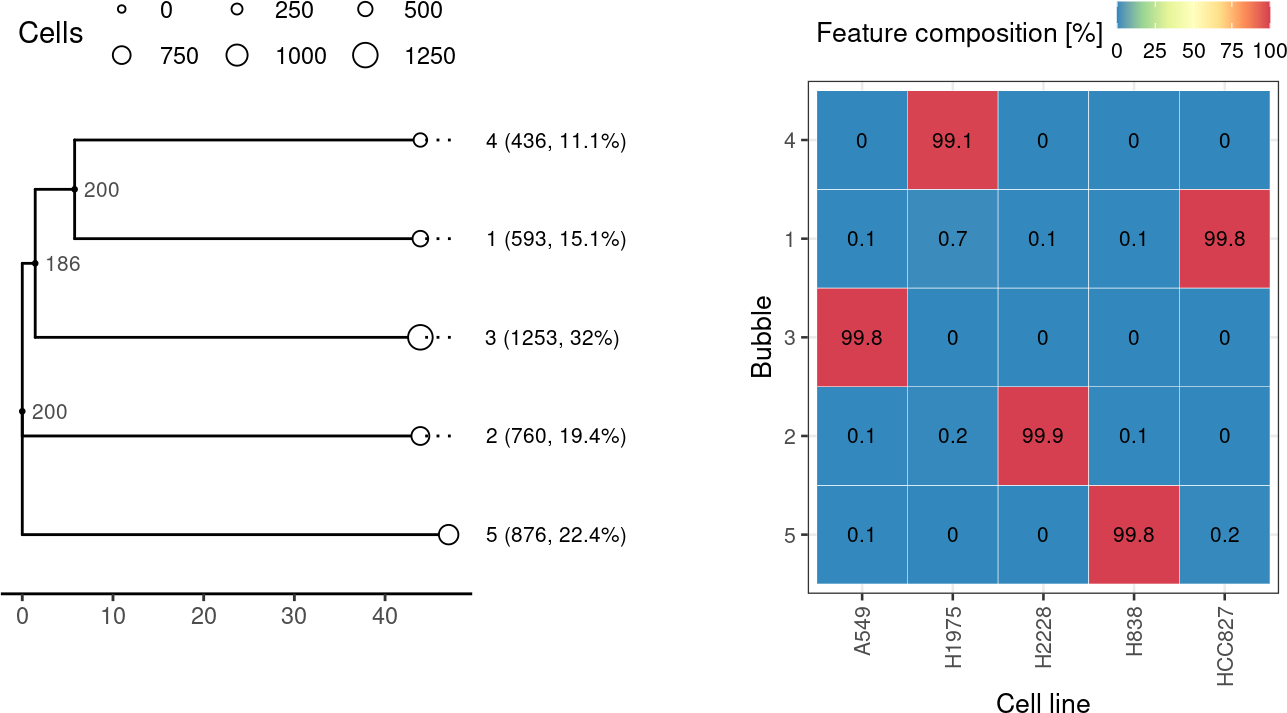We can also show the inter-bubble cell type composition, i.e. the relative frequencies of different cell types in a specific bubble (with parameter integrate_vertical=FALSE). Interpretation of the figure below: • the bubbles appear to be “pure” $$\rightarrow$$ made up of cells from distinct cell lines • the cell line composition of bubble 2 is: 0.1% H838, 99.9% H2228, 0.1% A549, 0.1% H1975 and 0% HCC827 cells • rows integrate to 100% w2 <- get_cat_tiles(btd = k5_kmeans, f = m$cell_line_demuxlet,
integrate_vertical = FALSE,
round_digits = 1,
x_axis_name = 'Cell line',
rotate_x_axis_labels = TRUE,
tile_text_size = 2.75)

(k5_kmeans$tree|w2$plot)+
patchwork::plot_layout(widths = c(1, 1))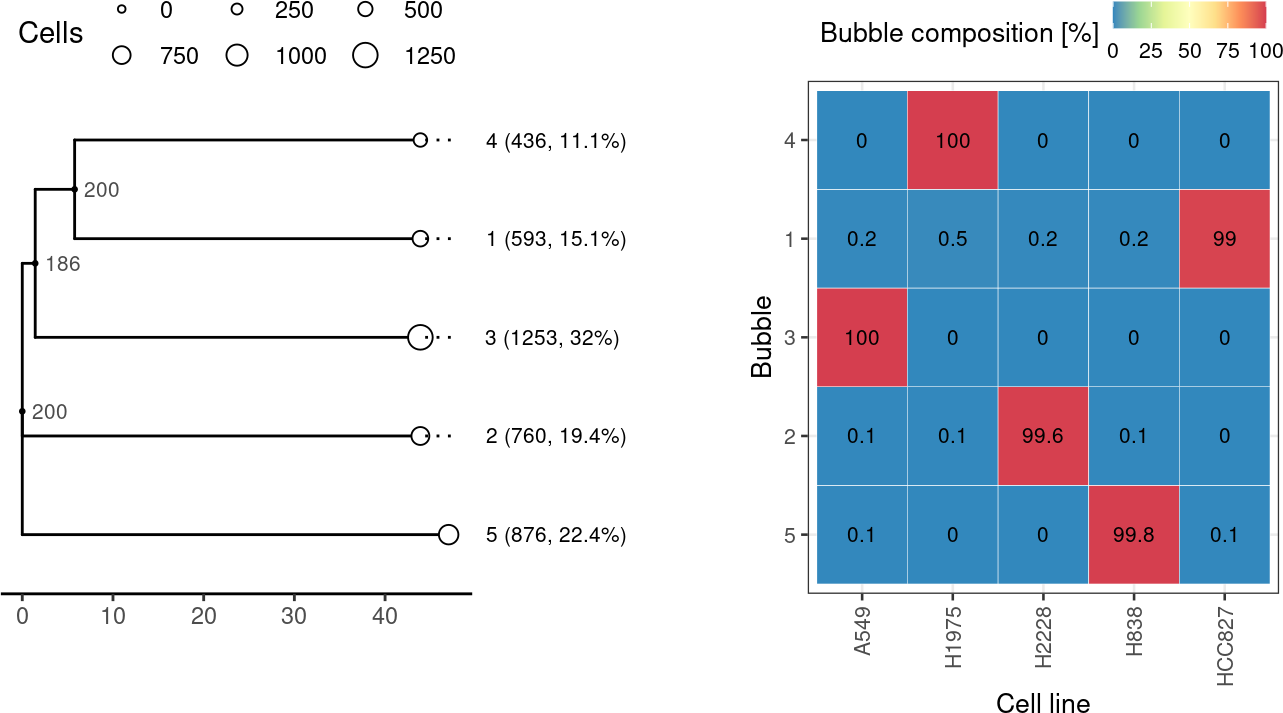scBubbletree uses R-package ggtree to visualize the bubbletree, and ggplot2 to visualize annotations. Furthermore, R-package patchwork is used to combine plots.

(k5_kmeans$tree|w1$plot|w2\$plot)+
patchwork::plot_layout(widths = c(1, 2, 2))+
patchwork::plot_annotation(tag_levels = "A")